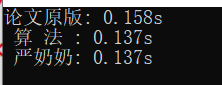# 神奇的快排（快排不同实现方法的性能差异）

## 性能对比《算法》中的快排比另外两个快了一半！

### 测试代码(一开始只是打算测试看看，解答自己的疑惑，所以命名比较随意，勿喷)：

#include <iostream>
#include <cstdlib>
#include <ctime>
using namespace std;
// 内联函数，要多次交换元素，调用次数多，防止函数调用开销影响最后结果
inline void swap(int* x, int* y) {
int temp = *x;
*x = *y;
*y = temp;
}

//////////// 严奶奶版本开始  ///////////
int partitionYan(int* arr, int low, int high) {
int p = arr[low];
while(low < high) {
while(low < high && arr[high] >= p)
high--;
arr[low] = arr[high];
while(low < high && arr[low] <= p)
low++;
arr[high] = arr[low];
}

arr[low] = p;

return low;
}

void QuickSortYan(int* A, int low, int high) {
int q;
if(low < high)
{
q = partitionYan(A, low, high);
QuickSortYan(A, low, q-1);
QuickSortYan(A, q+1, high);
}
}
//////////// 严奶奶版本结束  ///////////

//////////// 论文原版开始  ///////////
int Partition(int* A, int low, int high) {
int i = low, j = high;
// choos a bound
int bound = A[low];

while(1) {
// lower pointer start first, when the item equal to or less than bound, move upper
while(A[i] <= bound && i<=j) i++;
// then upper pointer start, when the ietm equal to or greater than bound, move lower
while(A[j] >= bound && i<=j) j--;

// The process continues until the pointers cross each other
if(i >= j)
break;
// exchange
swap(&A[i], &A[j]);
}

// The aim is now to produce a situation in
// which the keys of all items below a certain dividing line are equal to or less than the bound,
// while the keys of all items above the dividing line are equal to or greater than the bound.
// 所有元素交换完成后，要将 bound 放到两个 子数组的中间，以满足上述要求
A[low] = A[j];
A[j] = bound;

return j;
}

void QuickSort(int* A, int low, int high) {
int q;
if(low < high)
{
q = Partition(A, low, high);
QuickSort(A, low, q-1);
QuickSort(A, q+1, high);
}
}
//////////// 论文原版结束  ///////////

//////////// 《算法》版本开始 ///////////
int partition(int* a, int lo, int hi) {
int i = lo, j = hi + 1;
int v = a[lo];
while(true) {
while(a[++i] < v)
if(i == hi)
break;
while(a[--j] > v)
if( j == lo)
break;

if(i >= j) break;
swap(a[i], a[j]);
}
swap(a[lo], a[j]);
return j;
}

void QuickSortA(int* A, int low, int high) {
int q;
if(low < high)
{
q = partition(A, low, high);
QuickSortA(A, low, q-1);
QuickSortA(A, q+1, high);
}
}
//////////// 《算法》版本结束 ///////////

int main() {
clock_t start, end;
unsigned seed;
seed = time(0);
srand(seed);

int size = 1e7;

int* data1 = new int[size];
int* data2 = new int[size];
int* data3 = new int[size];
// 制造数据
for(int i = 0; i < size; i++) {
data1[i] = rand();
data2[i] = data1[i];
data3[i] = data1[i];
}
// QuickSort：论文原版
// QuickSortA：  算法第四版
// QuickSortYan： 严奶奶版
start = clock();
QuickSort(data1, 0, size-1);
end = clock();
cout<< "论文原版: " << (double)(end - start) / CLOCKS_PER_SEC << "s" << endl;

start = clock();
QuickSortA(data3, 0, size-1);
end = clock();
cout<< " 算 法 : " << (double)(end - start) / CLOCKS_PER_SEC<< "s" << endl;

start = clock();
QuickSortYan(data2, 0, size-1);
end = clock();
cout<< " 严奶奶: " << (double)(end - start) / CLOCKS_PER_SEC<< "s" << endl;

return 0;
}